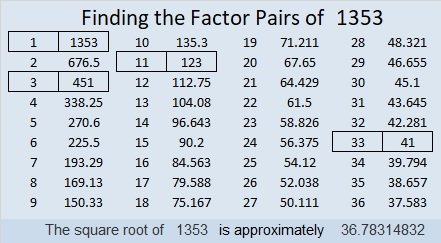# 1353 How to Solve a Level 4 Puzzle

Try solving this level 4 puzzle. If you need help with it, I explain the steps in the video below the puzzle:Print the puzzles or type the solution in this excel file: 10 Factors 1347-1356

Now I’ll tell you a little bit about the puzzle number, 1353:

• 1353 is a composite number.
• Prime factorization: 1353 = 3 × 11 × 41
• 1353 has no exponents greater than 1 in its prime factorization, so √1353 cannot be simplified.
• The exponents in the prime factorization are 1, 1, and 1. Adding one to each exponent and multiplying we get (1 + 1)(1 + 1)(1 + 1) = 2 × 2 × 2 = 8. Therefore 1353 has exactly 8 factors.
• The factors of 1353 are outlined with their factor pair partners in the graphic below.1353 is the hypotenuse of a Pythagorean triple:
297-1320-1353 which is 33 times (9-40-41)

This site uses Akismet to reduce spam. Learn how your comment data is processed.